# Probability + multiplication principle - math problems

#### Number of problems found: 52

• An aircraft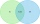An aircraft manufacturing company has submitted bids on two separate airline contracts, A and B. The company feels that it has a 70% chance of winning contract A and a 25% chance of winning contract B. Furthermore, it believes that winning contract A is i
• Right key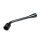The hostel has 4 rooms. The keys to each room are not numbered. Each of the four guests took one key. What is the probability that everyone took the right key?
• A marriedA married couple planned to have 3 children. i. List the possible combinations of the sexes of 3 children. Use B for boy and G for girl. ii. Calculate the probability that all three children would be of the same gender
• A bagA bag contains 9 blue marbles and 1 green marble. What is the probability of drawing a blue marble followed by a green marble, without replacing the first marble before drawing the second marble.
• Double six-sixWhat is the probability, that a player in the games of ludo will throw a double 6 twice .
• We rollWe roll two dice A. - what is the probability that the sum of the falling numbers is at most 4 B. - is at least 10 C. - is divisible by 5?
• Created trioWhat is the probability that in the created trio, which consists of 19 boys and 12 girls, they will be: a) the boys themselves b) the girls themselves c) 2 boys and 1 girl?
• Two diceWe roll two dice. What is the probability that the sum of the falling numbers is greater than 3?
• The box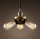The box contains three light bulbs with a wattage of 40 W and two pieces of light bulbs with a wattage of 60 W. What is the probability of the event that two randomly selected light bulbs will both be 40 W?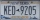A license plate has 3 letters followed by 4 numbers. Repeats are not allowed for the letters, but they are for the numbers. If they are issued at random, what is the probability that the 3 letters are in alphabetical order and the 3 numbers are consecutiv
• Three colors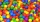Find the probability that 3 balls of the same color will be drawn from fate with 10 white, 10 red, and 10 blue balls.
• Hazard game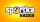In the Sportka hazard game, 6 numbers out of 49 are drawn. What is the probability that we will win: a) second prize (we guess 5 numbers correctly) b) the third prize (we guess 4 numbers correctly)?
• The box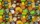The box contains five chocolate, three fruit, and two menthol candies. We choose sweets at random from the box. What probability will we take out one chocolate, one fruit, and one menthol candy without a return?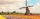How large must the group of people be so that the probability that two people have a birthday on the same day of the year is greater than 90%?
• Gold, silver, bronzeHow many ways can we divide gold, silver, bronze medals if 6 people are competing?
• The testThe test contains four questions, and there are five different answers to each of them, of which only one is correct, the others are incorrect. What is the probability that a student who does not know the answer to any question will guess the right answer
• Fall sum or sameFind the probability that if you roll two dice, it will fall the sum of 10, or the same number will fall on both dice.
• Birth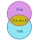Let's assume that the probability of the birth of a boy and a girl in the family is the same. What is the probability that in a family with five children, the youngest and oldest child is a boy?
• The universityAt a certain university, 25% of students are in the business faculty. Of the students in the business faculty, 66% are males. However, only 52% of all students at the university are male. a. What is the probability that a student selected at random in the
• Pairs of socks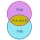Ferdinand has twelve pairs of socks, and one sock is leaky. What is the probability of putting on a leaky sock?

Do you have an exciting math question or word problem that you can't solve? Ask a question or post a math problem, and we can try to solve it.

We will send a solution to your e-mail address. Solved examples are also published here. Please enter the e-mail correctly and check whether you don't have a full mailbox.

Probability - math problems. Multiplication principle - math problems.